# The PANEL Procedure

### Specifying the Input Data

The PANEL procedure is similar to other regression procedures in SAS. Suppose you want to regress the variable Y on regressors X1 and X2. Cross sections are identified by the variable STATE, and time periods are identified by the variable DATE. The input data set used by PROC PANEL must be sorted by cross section and by time within each cross section. Therefore, the first step in PROC PANEL is to make sure that the input data set is sorted. The following statements sort the data set `A` appropriately:

```   proc sort data=a;
by state date;
run;
```

The next step is to invoke the PANEL procedure and specify the cross section and time series variables in an ID statement. The following statements shows the correct syntax:

```   proc panel data=a;
id state date;
model y = x1 x2;
run;
```

Alternatively, PROC PANEL has the capability to read "flat" data. Say that you are using the data set `A`, which has observations on states. Specifically, the data are composed of observations on
,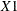, and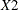. Unlike the previous case, the data is not recorded with a PROC PANEL structure. Instead, you have all of a state’s information on a single row. You have variables to denote the name of the state (say `state`). The time observations for thevariable are recorded horizontally. So the variable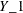is the first period’s time observation,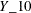is the tenth period’s observation for some state. The same holds for the other variables. You have variables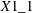to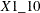,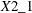to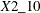, and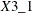to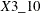for others. With such data, PROC PANEL could be called by using the following syntax:

```   proc panel data=a;
flatdata indid = state base = (Y X1 X2) tsname = t;
id state t;
model Y = X1 X2;
run;
```

See FLATDATA Statement and Example 20.2 for more information about the use of the FLATDATA statement.